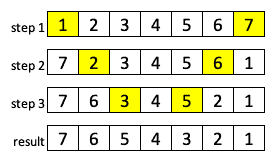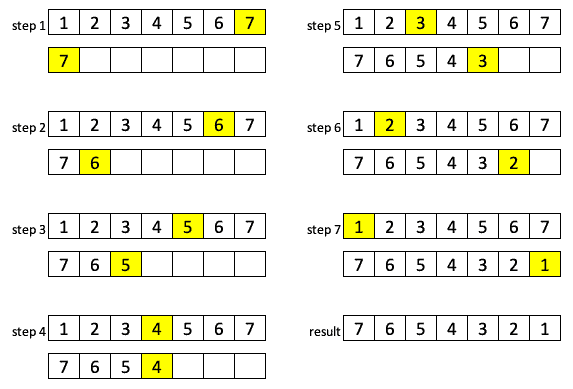## 1. Introduction

In this tutorial, we’ll explain how the in-place sorting algorithm works.

## 2. In-Place Algorithms

The in-place algorithms are those that don’t need any auxiliary data structure in order to transform the input data. Basically, it means that the algorithm doesn’t use extra space for input manipulation. It practically overrides the input with the output.

However, in reality, the algorithm actually may require a small and non-constant additional space for auxiliary variables. The complexity of this space is in most cases O(log n), although sometimes anything less than linear is allowed.

## 3. Pseudocode

Let’s now see some pseudocode and compare the in-place algorithm with the out-of-place one.

We’ll assume that we want to reverse an array of n numbers.

### 3.1. In-Place Algorithm

If we think about the problem, we’ll see that we have an input array and reversed array as the output. In the end, we don’t actually need our original array, only the reversed one.

Then, why wouldn’t we overwrite the input instead of moving its values to the completely new array, as it might look like a most obvious method? To do that, we’ll only need one additional variable to temporarily store the values that we’re currently working with:

``````reversInPlace(array A[n])
for i from 0 to n/2
temp = A[i]
A[i] = A[n - 1 - i]
A[n - 1 - i] = temp``````

It’s noteworthy to mention that no matter how big the array is, the extra space that we need will always be O(1) in this case.

The illustration shows that we need fewer steps than in the previous case:### 3.2. Out-of-Place Algorithm

On the other hand, we can also do this in a pretty simple, more obvious manner. We can create a new array of the same size, copy the values from the original one in the corresponding order and then delete the original array:

``````reverseOutOfPlace(array A[n])
create new array B[n]
for i from 0 to n - 1
B[i] = A[i]
delete A
return B``````

Although this will do what we wanted it to do, it’s not efficient enough. We have O(n) extra space required since we have two arrays to manipulate with. Besides that, creating and removing a new array is usually a slow operation.

Let’s see the illustration of the process:## 4. Java Implementation

Let’s now see how we can implement in Java what we learned in the previous section.

Firstly, we’ll implement an in-place algorithm:

``````public static int[] reverseInPlace(int A[]) {
int n = A.length;
for (int i = 0; i < n / 2; i++) {
int temp = A[i];
A[i] = A[n - 1 - i];
A[n - 1 - i] = temp;
}
return A;
}``````

We can test easily that this works as expected:

``````@Test
void givenArray_whenInPlaceSort_thenReversed() {
int[] input = {1, 2, 3, 4, 5, 6, 7};
int[] expected = {7, 6, 5, 4, 3, 2, 1};
assertArrayEquals("the two arrays are not equal", expected,
InOutSort.reverseInPlace(input));
}``````

Secondly, let’s check out the out-of-place algorithm implementation:

``````public static int[] reverseOutOfPlace(int A[]) {
int n = A.length;
int[] B = new int[n];
for (int i = 0; i < n; i++) {
B[n - i - 1] = A[i];
}
return B;
}``````

The test is pretty straightforward:

``````@Test
void givenArray_whenOutOfPlaceSort_thenReversed() {
int[] input = {1, 2, 3, 4, 5, 6, 7};
int[] expected = {7, 6, 5, 4, 3, 2, 1};
assertArrayEquals("the two arrays are not equal", expected,
InOutSort.reverseOutOfPlace(input));
}``````

## 5. Examples

There are many sorting algorithms that are using in-place approach. Some of them are insertion sort, bubble sort, heap sort, quicksort, and shell sort and you can learn more about them and check-out their Java implementations.

Also, we need to mention comb sort and heapsort. All these have space complexity O(log n).

It could be also useful to learn more about the Theory of Big-O Notation, as well as to check out some Practical Java Examples about the complexity of the algorithm.

## 6. Conclusion

In this article, we described the so-called in-place algorithms, illustrated how they work using pseudocode and a few examples, listed several algorithms that work on this principle, and finally implemented the basic examples in Java.

As usual, the entire code could be found over on GitHub.

### Get started with Spring and Spring Boot, through the Learn Spring course:

>> CHECK OUT THE COURSE